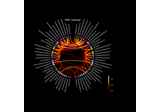# mne.viz.plot_connectivity_circle¶

mne.viz.plot_connectivity_circle(con, node_names, indices=None, n_lines=None, node_angles=None, node_width=None, node_colors=None, facecolor='black', textcolor='white', node_edgecolor='black', linewidth=1.5, colormap='hot', vmin=None, vmax=None, colorbar=True, title=None, colorbar_size=0.2, colorbar_pos=(- 0.3, 0.1), fontsize_title=12, fontsize_names=8, fontsize_colorbar=8, padding=6.0, fig=None, subplot=111, interactive=True, node_linewidth=2.0, show=True)[source]

Visualize connectivity as a circular graph.

Parameters
con`array`

Connectivity scores. Can be a square matrix, or a 1D array. If a 1D array is provided, “indices” has to be used to define the connection indices.

node_names

Node names. The order corresponds to the order in con.

indices

Two arrays with indices of connections for which the connections strengths are defined in con. Only needed if con is a 1D array.

n_lines

If not None, only the n_lines strongest connections (strength=abs(con)) are drawn.

node_angles`array`, shape (n_node_names,) | `None`

Array with node positions in degrees. If None, the nodes are equally spaced on the circle. See mne.viz.circular_layout.

node_width

Width of each node in degrees. If None, the minimum angle between any two nodes is used as the width.

node_colors

List with the color to use for each node. If fewer colors than nodes are provided, the colors will be repeated. Any color supported by matplotlib can be used, e.g., RGBA tuples, named colors.

facecolor`str`

Color to use for background. See matplotlib.colors.

textcolor`str`

Color to use for text. See matplotlib.colors.

node_edgecolor`str`

Color to use for lines around nodes. See matplotlib.colors.

linewidth`float`

Line width to use for connections.

colormap

Colormap to use for coloring the connections.

vmin

Minimum value for colormap. If None, it is determined automatically.

vmax

Maximum value for colormap. If None, it is determined automatically.

colorbarbool

Display a colorbar or not.

title`str`

The figure title.

colorbar_size`float`

Size of the colorbar.

colorbar_pos`tuple`, shape (2,)

Position of the colorbar.

fontsize_title`int`

Font size to use for title.

fontsize_names`int`

Font size to use for node names.

fontsize_colorbar`int`

Font size to use for colorbar.

padding`float`

Space to add around figure to accommodate long labels.

fig`None` | instance of `matplotlib.figure.Figure`

The figure to use. If None, a new figure with the specified background color will be created.

subplot`int` | `tuple`, shape (3,)

Location of the subplot when creating figures with multiple plots. E.g. 121 or (1, 2, 1) for 1 row, 2 columns, plot 1. See matplotlib.pyplot.subplot.

interactivebool

When enabled, left-click on a node to show only connections to that node. Right-click shows all connections.

node_linewidth`float`

Line with for nodes.

showbool

Show figure if True.

Returns
figinstance of `matplotlib.figure.Figure`

The figure handle.

axes

The subplot handle.

Notes

This code is based on a circle graph example by Nicolas P. Rougier

By default, `matplotlib.pyplot.savefig()` does not take `facecolor` into account when saving, even if set when a figure is generated. This can be addressed via, e.g.:

```>>> fig.savefig(fname_fig, facecolor='black')
```

If `facecolor` is not set via `matplotlib.pyplot.savefig()`, the figure labels, title, and legend may be cut off in the output figure.

## Examples using `mne.viz.plot_connectivity_circle`¶